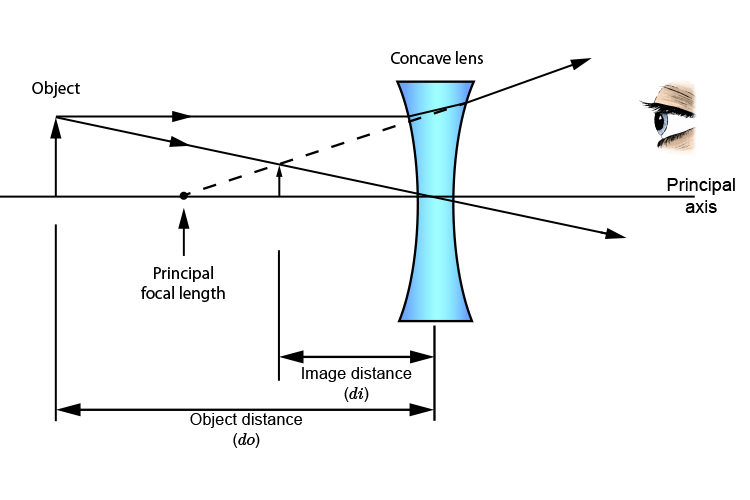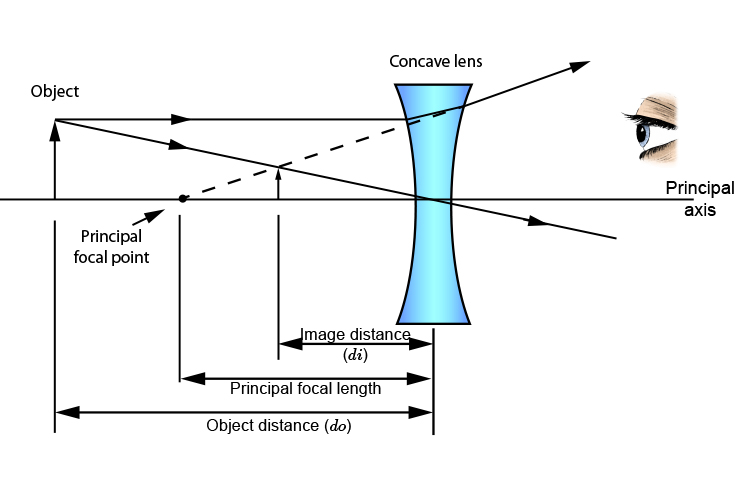# Object distance and image distance – Concave lens

The object distance is the distance from the object to the centre of the lens. This is denoted by the symbol do.

The image distance (sometimes confused with the focal length) is the distance from the image to the centre line of the lens. This is denoted by the symbol di.With the principal focal length added, this would look like: